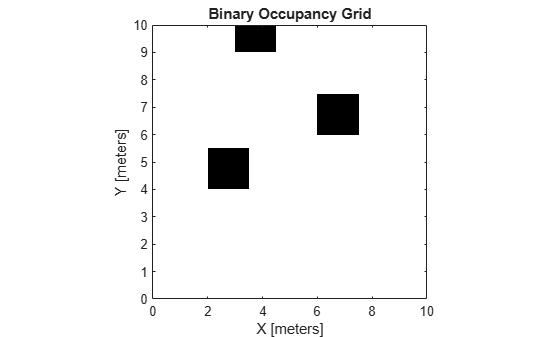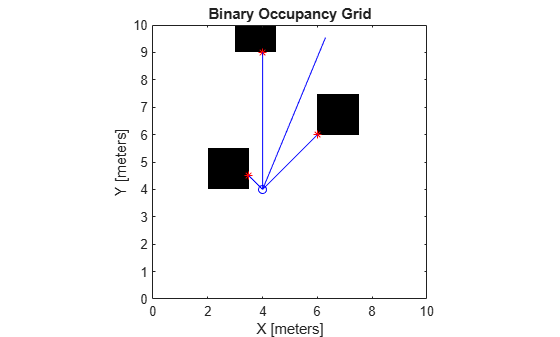# rayIntersection

Find intersection points of rays and occupied map cells

Since R2019b

## Syntax

``intersectionPts = rayIntersection(map,pose,angles,maxrange)``

## Description

example

````intersectionPts = rayIntersection(map,pose,angles,maxrange)` returns intersection points of rays and occupied cells in the specified `map`. Rays emanate from the specified `pose` and `angles`. Intersection points are returned in the world coordinate frame. If there is no intersection up to the specified `maxrange`, `[NaN NaN]` is returned.```

## Examples

collapse all

Create a binary occupancy grid map. Add obstacles and inflate them. A lower resolution map is used to illustrate the importance of the size of your grid cells. Show the map.

```map = binaryOccupancyMap(10,10,2); obstacles = [4 10; 3 5; 7 7]; setOccupancy(map,obstacles,ones(length(obstacles),1)) inflate(map,0.25) show(map)```Find the intersection points of occupied cells and rays that emit from the given vehicle pose. Specify the max range and angles for these rays. The last ray does not intersect with an obstacle within the max range, so it has no collision point.

```maxrange = 6; angles = [pi/4,-pi/4,0,-pi/8]; vehiclePose = [4,4,pi/2]; intsectionPts = rayIntersection(map,vehiclePose,angles,maxrange)```
```intsectionPts = 4×2 3.5000 4.5000 6.0000 6.0000 4.0000 9.0000 NaN NaN ```

Plot the intersection points and plot rays from the pose to the intersection points.

```hold on plot(intsectionPts(:,1),intsectionPts(:,2),'*r') % Intersection points plot(vehiclePose(1),vehiclePose(2),'ob') % Vehicle pose for i = 1:3 plot([vehiclePose(1),intsectionPts(i,1)],... [vehiclePose(2),intsectionPts(i,2)],'-b') % Plot intersecting rays end plot([vehiclePose(1),vehiclePose(1)-6*sin(angles(4))],... [vehiclePose(2),vehiclePose(2)+6*cos(angles(4))],'-b') % No intersection ray```## Input Arguments

collapse all

Map representation, specified as a `binaryOccupancyMap` object. This object represents the environment of the robot. The object contains a matrix grid with binary values indicating obstacles as `true` (`1`) and free locations as `false` (`0`).

Position and orientation of the sensor, specified as an ```[x y theta]``` vector. The sensor pose is an x and y position with angular orientation theta (in radians) measured from the x-axis.

Ray angles emanating from the sensor, specified as a vector with elements in radians. These angles are relative to the specified sensor `pose`.

Maximum range of laser range sensor, specified as a scalar in meters. Range values greater than or equal to `maxrange` are considered free along the whole length of the ray, up to `maxrange`.

## Output Arguments

collapse all

Intersection points, returned as n-by-2 matrix of ```[x y]``` pairs in the world coordinate frame, where n is the length of `angles`.

## Version History

Introduced in R2019b